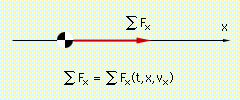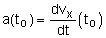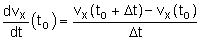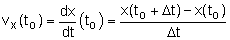Ch 2. Particle Force and Acceleration Multimedia Engineering Dynamics Rect.Coord. Normal/Tang. Coord. PolarCoord. Orbital Mechanics Computational Mechanics
 Chapter - Particle - 1. General Motion 2. Force & Accel. 3. Energy 4. Momentum - Rigid Body - 5. General Motion 6. Force & Accel. 7. Energy 8. Momentum 9. 3-D Motion 10. Vibrations Appendix Basic Math Units Basic Equations Sections Search eBooks Dynamics Fluids Math Mechanics Statics Thermodynamics Author(s): Kurt Gramoll ©Kurt GramollDYNAMICS - THEORY Until now, the motion of an object was determined using the simple procedure, Use Newton's Second Law to determine the acceleration, then... Integrate to find analytical (closed-form) solutions for velocity and acceleration. In many situations, the functions describing the force (and thus the acceleration) are too complicated to integrate and find closed-form solutions. Approximate solutions can be obtained using numerical integration. Finite-Difference MethodStraight Line Motion The finite-difference method is a method of numerical integration that determines changes in dependent variables over finite intervals of time. Consider an object of mass m in straight-line motion along the x-axis. The force acting on the object may depend on time, position, and velocity. The corresponding acceleration will also be a function of time, position, and velocity, and thus cannot easily be integrated to find velocity and position.      a = F/m = 1/m ΣFx (t,x,vx)                            (1) Suppose that at a particular time, to, the position x(to) and velocity vx(to) is known. By definition, the acceleration of the object at to is= 1/m ΣFx(to, x(to), vx(to))                    (2) The time derivative of vx at to can be approximated asSubstituting this into Eq. 2 gives an approximate expression for the velocity at to + Δt as      vx(to + Δt) =  vx(to)                        + 1/m ΣFx(to, x(to), vx(to))Δt        (3) The relationship between the velocity and position can be approximated asFrom this an approximate expression for the position at time to + Δt can be determined as      x(to + Δt) = x(to) + vx(to) Δt                          (4) Thus, if the position and velocity are known at time to, then their values can be approximated at to + Δt by using Eqs. 3 and 4. The procedure can then be repeated using x(to + Δt) and v(to + Δt) as the initial conditions to determine the approximate position and velocity at to + 2 Δt. Continuing in this fashion, an approximate solution for the position and velocity can be obtained in terms of time. Because of the number of calculations required, this process is greatly simplified with the use of a computer. Curvilinear Motion The position and velocity of an object in curvilinear motion can also be determined using the same approach. If an object moves in the x-y plane, the components of force may depend on time, position, and velocity,      ΣFx = ΣFx(t,x,y,vx,vy)      ΣFy = ΣFy(t,x,y,vx,vy) If the position and time are known at time to, a derivation similar to that for Eqs. 3 and 4 can be used to find the position and velocity at t + Δt.      x(to + Δt) = x(to) + vx(to) Δt                          (5a)      y(to + Δt) = y(to) + vy(to) Δt                          (5b) vx(to + Δt) =  vx(to) + (1/m) ΣFx(to, x(to), y(to), vx(to), vy(to))Δt         (5c)                                       vy(to + Δt) =  vy(to) + (1/m) ΣFy(to, x(to), y(to), vx(to), vy(to))Δt         (5d)

Practice Homework and Test problems now available in the 'Eng Dynamics' mobile app
Includes over 400 problems with complete detailed solutions.
Available now at the Google Play Store and Apple App Store.